Test: Motion: 1

# Test: Motion: 1

Test Description

## 25 Questions MCQ Test Science Class 9 | Test: Motion: 1

Test: Motion: 1 for Class 9 2023 is part of Science Class 9 preparation. The Test: Motion: 1 questions and answers have been prepared according to the Class 9 exam syllabus.The Test: Motion: 1 MCQs are made for Class 9 2023 Exam. Find important definitions, questions, notes, meanings, examples, exercises, MCQs and online tests for Test: Motion: 1 below.
Solutions of Test: Motion: 1 questions in English are available as part of our Science Class 9 for Class 9 & Test: Motion: 1 solutions in Hindi for Science Class 9 course. Download more important topics, notes, lectures and mock test series for Class 9 Exam by signing up for free. Attempt Test: Motion: 1 | 25 questions in 25 minutes | Mock test for Class 9 preparation | Free important questions MCQ to study Science Class 9 for Class 9 Exam | Download free PDF with solutions
 1 Crore+ students have signed up on EduRev. Have you?
Test: Motion: 1 - Question 1

### Which of the following can determine the acceleration of a moving object?

Detailed Solution for Test: Motion: 1 - Question 1

The slope of the velocity-time graph determines the acceleration.

Test: Motion: 1 - Question 2

### Equal and opposite forces acting on a body which do not change its state of rest or uniform motion are called:

Detailed Solution for Test: Motion: 1 - Question 2

Balanced forces are two forces acting in opposite directions on an object, and equal in size. Anytime there is a balanced force on an object, the object stays still or continues moving continues to move at the same speed and in the same direction.

Test: Motion: 1 - Question 3

### Find the average speed of a bicycle if it completes two round of a circular track of radius 140m twice in 5min 52 sec.

Detailed Solution for Test: Motion: 1 - Question 3

Radius of truck = r = 140m
circumfarance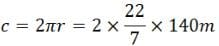Time taken to cover the distance = t = 5 min 52 sec = 352 sec
Since it complete 2 rounds, therefor distance it travels is 2 times of circumference.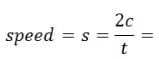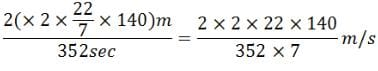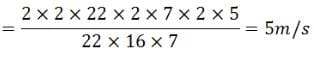Test: Motion: 1 - Question 4

Force of friction always acts in the direction:

Detailed Solution for Test: Motion: 1 - Question 4

Friction is a resisting force that acts on a body to prevent or retard its motion. Friction force always acts tangent to the surface at points of contact. Friction force acts opposite to the direction of motion.

Test: Motion: 1 - Question 5

When balanced forces act on a body, the body:

Detailed Solution for Test: Motion: 1 - Question 5

Forces are said to be balanced when two equal and opposite forces acts on an object which makes net force to be zero. Balanced forces are acting means object will be rest. When unbalanced forces are acting on object it starts moving in the direction of force. Example a book kept on table has two forces gravitational force acting downwards and normal force acting upwards. As two forces are equal and opposite the net force will be zero and object remains at rest.

So option D is correct

Test: Motion: 1 - Question 6

When unbalanced forces act on a body, the body:

Detailed Solution for Test: Motion: 1 - Question 6

The correct answer is C as When unbalanced forces act on a body, the body: as it must experience acceleration by the force applied on it.

Test: Motion: 1 - Question 7

If no external force acts on a body, it will :

Detailed Solution for Test: Motion: 1 - Question 7

Torque is the rotational analogue of force and angular velocityis the rotational analogue of linear velocity in rotational mechanics and when no external force act on the body, its velocity is constant. similarly when no torque acts on a body, its angular velocity is constant.

So option D is correct.

Test: Motion: 1 - Question 8

If a body is allowed to freely fall from a height, its speed increases continuously. It is because:

Detailed Solution for Test: Motion: 1 - Question 8

The acceleration mentioned is due to the force of gravity. This is a force of attraction based on the mass of an object and the distance between the two objects Newton's law of universal gravitation. Generally on the surface of the earth we treat this as 9.8 m/sec^2 or 32 ft/sec^2. The way that Einstein describes it is that mass curves spacetime, thus space itself is pushing down on the falling object and is the cause of the force

So option C is correct.

Test: Motion: 1 - Question 9

A force of magnitude' F' acts on a body of mass 'm'. The acceleration of the body depends upon:

Detailed Solution for Test: Motion: 1 - Question 9

Mass is both a property of a physical body and a measure of its resistance to acceleration (a change in its state of motion) when a net force is applied. It also determines the strength of its mutual gravitational attraction to other bodies. The basic SI unit of mass is the kilogram (kg).

Test: Motion: 1 - Question 10

Force remaining constant, if the mass of body increases, its acceleration is likely to :

Detailed Solution for Test: Motion: 1 - Question 10

If the degree of force acting on the object is the same ( F = ma) , then as mass increases, acceleration will go down ( a = F / m) .
So, option ( c) decrease is the correct answer.

Test: Motion: 1 - Question 11

A force F produces an acceleration 'a' in a body. The same force produces and acceleration 4a in another body. The mass of other body is :

Detailed Solution for Test: Motion: 1 - Question 11

GIVEN:-
FORCE PRODUCED IN THE BODY =  F
ACCELERATION PRODUCED WITH FORCE F =  a
ACCELERATION PRODUCED IN OTHER BODY BY SAME FORCE = 4a

To find : Mass of the other body where acceleration produced is 4a

First body -
F = m * a
f/a = m  --------- (eq.01)

Second body -
Let mass be x
F = X * 4a
F/4a = X   ------(eq.02)

Solving the equation we get-
X = ¼ m

Test: Motion: 1 - Question 12

When a running motorbike accelerates suddenly, the pillion rider has a tendency to fall backward. This is an example of:

Detailed Solution for Test: Motion: 1 - Question 12

Newton's first law of motion follows that if the body is in rest or moving with the uniform velocity it will be in rest or uniform velocity unless or until an external force is applied on the body to change its state of motion. So when the bike suddenly accelerates the body of pillion is not able to make the body accelerate so fast because when the bike was running his body was also moving with that motion only. So when the bike suddenly accelerates it was not able to move with that acceleration.

Test: Motion: 1 - Question 13

Which of the following is a vector quantity?
A. Retardation
B. Speed
C. Force
D. Distance

Detailed Solution for Test: Motion: 1 - Question 13

"Vector quantities have both magnitude and direction. Scalar quantities have only magnitude."

Test: Motion: 1 - Question 14

Which of the is true for displacement?
A. It cannot be Zero
B. Its magnitude is greater than the distance travelled by the object
C. It’s the shortest distance between initial & final position
D. It is a vector quantity

Detailed Solution for Test: Motion: 1 - Question 14

The displacement is a vector quantity (magnitude and direction) that describes the difference between the final and initial positions of a moving object. It is the shortest distance moved in a certain direction. The displacement is the vector AD whose magnitude if 5 km and its direction is to the east.

Test: Motion: 1 - Question 15

Statement A: When a body moves with uniform speed, then the average speed is same as instantaneous speed.
Statement B: Average speed is less than average velocity.
Which of the above statement is true

Detailed Solution for Test: Motion: 1 - Question 15 If the runner starts and finishes the race at the same point, then the overall displacement will be zero. When a body moves with uniform speed, then the average speed is same as instantaneous speed. Average speed is calculated by dividing the total distance travelled with total time taken.

The instantaneous speed of an object can also be determined through calculating the average speed over a short distance and time. Summary: “
Constant speed” means an object is moving at the same speed throughout. ... At a certain instant, what the speedometer reads is the instantaneous speed.

Test: Motion: 1 - Question 16

A car is moving with uniform speed and covers 100m in 50 second find the speed.

Detailed Solution for Test: Motion: 1 - Question 16

SPEED = DISTANCE / TIME
DISTANCE = 100M
TIME = 50 SEC
THEN, SPEED =100M / 50 SEC
SPEED = 2 M / S

Test: Motion: 1 - Question 17

Instantaneous speed of a vehicle is measured by

Detailed Solution for Test: Motion: 1 - Question 17

Speedometer is device that measure the speed of vehicle, at any instant of time i.e measure instantaneous speed.

Test: Motion: 1 - Question 18

A particle covers equal distance in equal interval of time. It is said to be

Detailed Solution for Test: Motion: 1 - Question 18

Speed is a scalar quantity which means it has only magnitude and no direction, btw velocity is a vector quantity which means it has both magnitude and no direction.

It's safe to say that the answer is uniform speed because the question didn't mention about the particle covering equal distances in equal intervals of time in a particular direction. Therefore, the answer is uniform speed.

Had the question have mentioned 'in a particular direction' then the answer would have been uniform velocity.

Therefore, the answer is UNIFORM SPEED.

Test: Motion: 1 - Question 19

Statement A: A curved line in a speed – time graph means non-uniform motion.
Statement B : A curved line in a velocity – time graph means uniform acceleration

Detailed Solution for Test: Motion: 1 - Question 19

A curved line in a speed - time graph means non - uniform motion. In speed - time graph, uniform motion is represented by straight line parallel to time zone . We know that, slope of the velocity - time graph gives the acceleration of the body. If the velocity Time graph is a curved line, this means it's slope changes at every instant. Thus the body is said to be in non - uniform motion.

So option A is correct

Test: Motion: 1 - Question 20

A train 120m long moving on a straight and level track with uniform speed passes a pole in 6 seconds. Find the time it will take to cross a 50m long bridge.

Detailed Solution for Test: Motion: 1 - Question 20

AS, d=120m (length of train)

t=6 sec (to pass the pole)

v=?

Therefore,

v=d\t

=120\6

=20m\s

As, The train is 120 m long and bridge is 50 m long = {120+ 50 = 170 m }                                                                                    So, Time taken = distance/speed

= 170/20

= 8.5 seconds

Test: Motion: 1 - Question 21

Match the following with correct response.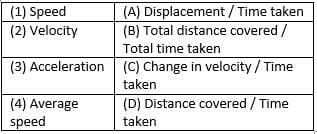Detailed Solution for Test: Motion: 1 - Question 21

The correct option is B.
For a body moving at a uniform velocity you can calculate the speed by dividing the distance traveled by the amount of time it took, for example one mile in 1/2 hour would give you 2 miles per hour.
In order to find the velocity of the moving object, you will need to divide the change in position by the change in time. Specify the direction moved, and you have the average velocity.
Calculating acceleration involves dividing velocity by time — or in terms of SI units, dividing the meter per second [m/s] by the second [s]. Dividing distance by time twice is the same as dividing distance by the square of time. Thus the SI unit of acceleration is the meter per second squared
Average speed is calculated by dividing distance by time (e.g. miles/hour). Okay we're going to talk about average speed, instantaneous speed is the distance that you're covering at a specific time. So 100 kilometers an hour, average speed is the total distance you're going to cover over a period of time.

Test: Motion: 1 - Question 22

Which of the following is an example of uniform motion?
A. The motion of car in the busy road
B. The motion of a satellite around the planet
C. The motion of moon around the earth
D. The motion of any particle on the arm of a clock

Detailed Solution for Test: Motion: 1 - Question 22

Satellites revolve around their planets in almost circular orbits with constant speed. Thus, during their motion, the speed remains constant, while the direction of motion changes continuously. As a result, there is a change in their velocity. Moon moves in uniform circular motion around the earth. It is the force of gravity of the earth which keeps the moon in circular orbit around it. The motion of any particle on the arm of a clock is circular as thus uniform motion.

Test: Motion: 1 - Question 23

Which of the following physical quantity is not present in first equation of motion?

Detailed Solution for Test: Motion: 1 - Question 23

first equation of motion is : v = u + at

Test: Motion: 1 - Question 24

Which of the following is the case of accelerated motion?

Detailed Solution for Test: Motion: 1 - Question 24

Case of Accelerated Motion
Uniform Acceleration

A body under constant acceleration is uniformly accelerated motion. This is when a constant external force is applied to the body. The direction of acceleration is very important in the change of velocity. However, the direction of acceleration of a body may not be same as the direction of its motion. For example, if a car travels at a speed of 60 km/hour then it will cover a distance of 1 km/minute. Here the motion of car acceleration is uniform.

Motion is an athlete running 100m along a straight track. Linear motion is the most basic of all motion. According to Newton's first law of motion, objects that do not experience any net force will continue to move in a straight line with a constant velocity until they are subjected to a net force.

So option D is correct.

Test: Motion: 1 - Question 25

What is the quantity which is measured as the area occupied in the velocity-time graph?

Detailed Solution for Test: Motion: 1 - Question 25

The area under the velocity time graph will give us the displacement of an object and the slope gives the acceleration

we should find the area under the graph , if the graph is irregular we need to split it into parts and finally add them and if the shape is not at all regular we should go for integration

## Science Class 9

115 videos|302 docs|163 tests
 Use Code STAYHOME200 and get INR 200 additional OFF Use Coupon Code
Information about Test: Motion: 1 Page
In this test you can find the Exam questions for Test: Motion: 1 solved & explained in the simplest way possible. Besides giving Questions and answers for Test: Motion: 1, EduRev gives you an ample number of Online tests for practice

## Science Class 9

115 videos|302 docs|163 tests